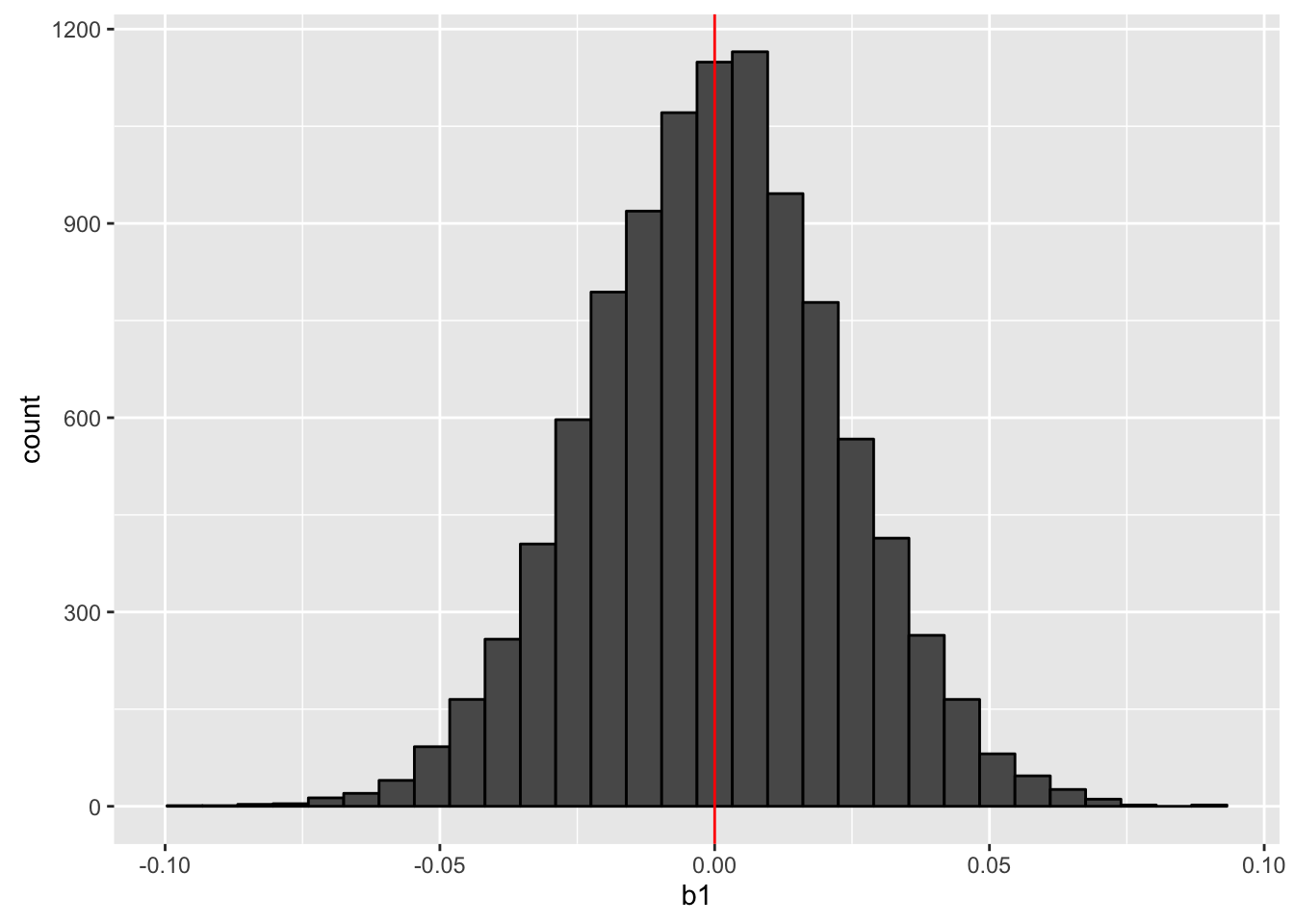## Preparation

We need some packages to simulate clustered data analysis. Let us load them here. Install them before loading if necessary by install.packages().

library('arm')
library('mvtnorm')
library('lme4')
library('multiwayvcov')
library('clusterSEs')
library('ggplot2')
library('dplyr')
library('haven')

## Simulations of Linear Regression

### Setting Up Simulations

First, let us create a function to create data. Here we suppose a simple regression model: $y_i \sim \mbox{N}(\beta_0 + \beta_1 x_i, \sigma^2).$

In the fucntion, intra-cluster correlation is set by rho ($$\rho$$). When $$\rho = 1$$, all units within a cluster are cosidered to be identical, and the effective sample size is reduced to the number of clusters. When $$\rho =0$$, there is no correlation of units within a cluster, and all observations are considered to be independent of each other.

gen_cluster <- function(param = c(.1, .5), n = 1000, n_cluster = 50, rho = .5) {
# Function to generate clustered data
# Required package: mvtnorm

# individual level
Sigma_i <- matrix(c(1, 0, 0, 1 - rho), ncol = 2)
values_i <- rmvnorm(n = n, sigma = Sigma_i)

# cluster level
cluster_name <- rep(1:n_cluster, each = n / n_cluster)
Sigma_cl <- matrix(c(1, 0, 0, rho), ncol = 2)
values_cl <- rmvnorm(n = n_cluster, sigma = Sigma_cl)

# predictor var consists of individual- and cluster-level components
x <- values_i[ , 1] + rep(values_cl[ , 1], each = n / n_cluster)

# error consists of individual- and cluster-level components
error <- values_i[ , 2] + rep(values_cl[ , 2], each = n / n_cluster)

# data generating process
y <- param + param*x + error

df <- data.frame(x, y, cluster = cluster_name)
return(df)
}

Next, let us make some functions to run simulations.

# Simulate a dataset with clusters and fit OLS
# Calculate cluster-robust SE when cluster_robust = TRUE
cluster_sim <- function(param = c(.1, .5), n = 1000, n_cluster = 50,
rho = .5, cluster_robust = FALSE) {
# Required packages: mvtnorm, multiwayvcov
df <- gen_cluster(param = param, n = n , n_cluster = n_cluster, rho = rho)
fit <- lm(y ~ x, data = df)
b1 <- coef(fit)
if (!cluster_robust) {
Sigma <- vcov(fit)
se <- sqrt(diag(Sigma))
b1_ci95 <- confint(fit)[2, ]
} else { # cluster-robust SE
Sigma <- cluster.vcov(fit, ~ cluster)
se <- sqrt(diag(Sigma))
t_critical <- qt(.025, df = n - 2, lower.tail = FALSE)
lower <- b1 - t_critical*se
upper <- b1 + t_critical*se
b1_ci95 <- c(lower, upper)
}
return(c(b1, se, b1_ci95))
}

# Function to iterate the simulation. A data frame is returned.
run_cluster_sim <- function(n_sims = 1000, param = c(.1, .5), n = 1000,
n_cluster = 50, rho = .5, cluster_robust = FALSE) {
# Required packages: mvtnorm, multiwayvcov, dplyr
df <- replicate(n_sims, cluster_sim(param = param, n = n, rho = rho,
n_cluster = n_cluster,
cluster_robust = cluster_robust))
df <- as.data.frame(t(df))
names(df) <- c('b1', 'se_b1', 'ci95_lower', 'ci95_upper')
df <- df %>%
mutate(id = 1:n(),
param_caught = ci95_lower <= param & ci95_upper >= param)
return(df)
}

### Data without Clusters

First, we will run simulations of data with no cluster ($$\rho=0$$). Here, we set $$\beta_0 = 0.4$$ and $$\beta_1 = 0$$. Setting the significance level at 5%, we should incorrectly reject the null $$\beta_1 = 0$$ in about 5 percent of the simulations.

sim_params <- c(.4, 0)   # beta1 = 0: no effect of x on y
sim_nocluster <- run_cluster_sim(n_sims = 10000, param = sim_params, rho = 0)
hist_nocluster <- ggplot(sim_nocluster, aes(b1)) +
geom_histogram(color = 'black') +
geom_vline(xintercept = sim_params, color = 'red')
print(hist_nocluster)As the histogram above shows, we can estimate the value of $$\beta_1$$ correctly on average. Let’s check the confidence intervals (CIs).

ci95_nocluster <- ggplot(sample_n(sim_nocluster, 100),
aes(x = reorder(id, b1), y = b1,
ymin = ci95_lower, ymax = ci95_upper,
color = param_caught)) +
geom_hline(yintercept = sim_params, linetype = 'dashed') +
geom_pointrange() +
labs(x = 'sim ID', y = 'b1', title = 'Randomly Chosen 100 95% CIs') +
scale_color_discrete(name = 'True param value', labels = c('missed', 'hit')) +
coord_flip()
print(ci95_nocluster)